YOU CAN CODE!With The Case Of UCanCode.net  Release The Power OF  Visual C++ ! Home |  Products | Purchase |  Support | Downloads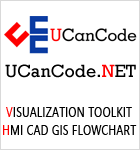View in English
View in Japanese
View in
참고
View in Français
View in Italiano
View in 中文(繁體)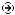Download EvaluationPricing & Purchase?E-XD++Visual C++/ MFC ProductsOverviewElectronic Form SolutionVisualization & HMI SolutionPower system HMI SolutionBar code labeling SolutionWorkflow SolutionCoal industry HMI SolutionInstrumentation Gauge SolutionReport Printing SolutionGraphical modeling SolutionGIS mapping solutionVisio graphics solutionIndustrial monitoring SolutionFlowchart and diagramming SolutionOrganization Diagram SolutionGraphic editor Source CodeUML drawing editor Source CodeMap Diagramming SolutionArchitectural Graphic Drawing SolutionRequest EvaluationVX++ Cross-Platform C/C++OverviewActiveX COM ProductsOverviewTechnical Support# WPF 3D Article, Tutorial with Chart Graphics C# Code.

## 1

When using WPF for 3D graphics, many people have concerns over the performance. Following the guidelines from Microsoft online help, I built a 3D surface chart, as shown in the picture above. The surface chart has more than 40,000 vertices and more than 80,000 triangles. The performance is still fine. The project also includes 3D scatter plot which has a large number of data points. You can build the project, feel the performance of WPF 3D and decide whether WPF 3D is suitable for your 3D data visualization.

## 2

This section briefly goes through the steps for building 3D graphics using WPF. Although there are many tutorials on WPF 3D, I still give a brief review here to help understand the class structure of this project.

The WPF 3D is displayed within the `Viewport3D` UI elements. The three basic components are:

• Camera
• Light
• 3D model

For 3D chart, we are not concerned too much about the camera and light. Those properties are set in the XAML file, as shown below. The 3D model will be set in C# code.

```<Window x:Class="WPF Chart.Window1"
xmlns="http://schemas.microsoft.com/winfx/2006/xaml/presentation"
xmlns:x="http://schemas.microsoft.com/winfx/2006/xaml"
Title="WPF 3D Chart" Height="500" Width="600">
<Grid>
<Viewport3D Name="mainViewport" >
<Viewport3D.Camera>
<OrthographicCamera x:Name="camera"
FarPlaneDistance="10"
NearPlaneDistance="1"
LookDirection="0,0,-1"
UpDirection="0,1,0"
Position="0,0,2" />
</Viewport3D.Camera>
<Viewport3D.Children>
<ModelVisual3D x:Name="Light1">
<ModelVisual3D.Content>
<DirectionalLight Color="White" Direction="1,1,-1"/>
</ModelVisual3D.Content>
</ModelVisual3D>
</Viewport3D.Children>
</Viewport3D>
</Grid>
</Window>```

The root element is a `Window`. Inside the `window`, we use `Grid` layout. Those two elements are provided by Visual Studio when we build the project. Inside the `grid`, we add a `Viewport3D` to hold the 3D object. Under the`Viewport3D`, we have a camera, a directional light.

We added the camera and light in the XAML file. Now, we add the 3D model in C# code. The mesh structure (type`System.Windows.Media.Media3D.MeshGeometry3D`) consists of four parts of data:

1. Vertices location
2. Connection between vertices
3. Normal direction of vertices
4. Texture mapping coordinate of each vertex

The vertices location is represented by a `Point3D` structure.

```System.Windows.Media.Media3D.Point3D point0 = new Point3D(-0.5, 0, 0);
System.Windows.Media.Media3D.Point3D point1 = new Point3D(0.5, 0.5, 0.3);
System.Windows.Media.Media3D.Point3D point2 = new Point3D(0, 0.5, 0);```

Those points are put into `Positions` array of the mesh structure.

```System.Windows.Media.Media3D.MeshGeometry3D triangleMesh = new MeshGeometry3D();

Three vertices make a triangle. The vertices connections are described by three integers, which are the indices of the 3 vertices in the `Positions` array.

```int n0 = 0;
int n1 = 1;
int n2 = 2;```

The 3 indices of a triangle are added to the `TriangleIndices` array.

```triangleMesh.TriangleIndices.Add(n0);

The order of the indices decides whether the triangle is front surface or back surface. The front surface and back surface usually have different properties. The WPF 3D display also needs to know the normal direction of the vertices.

```System.Windows.Media.Media3D.Vector3D norm = new Vector3D(0, 0, 1);

We will discuss the texture mapping in a later section. The above code only shows one triangle. By combining many triangles, we can get a mesh structure. Now, we will attach material properties to the mesh surface.

```System.Windows.Media.Media3D.Material frontMaterial =
new DiffuseMaterial(new SolidColorBrush(Colors.Blue));```

Combining mesh and material, we can get a 3D model.

```System.Windows.Media.Media3D.GeometryModel3D triangleModel =
new GeometryModel3D(triangleMesh, frontMaterial);```

The `GeometryModel3D `object also has a `transform `property. We will discuss it in the next section.

`triangleModel.Transform = new Transform3DGroup();`

The 3D model we created will be attached to a visual element:

```System.Windows.Media.Media3D.ModelVisual3D visualModel = new ModelVisual3D();
visualModel.Content = triangleModel;```

The `ModelVisual3D` object will be displayed in `Viewport3D`:

`this.mainViewport.Children.Add(visualModel);`

This involves quite a lot of steps. `Model3D` class in this project helps to generate a `ModelVisual3D` object. If we run the program, we will see a blue triangle. We cannot rotate it yet. In the next section, we will show how to rotate this 3D model.

## 3

In this section, we will use the mouse to rotate the 3D model. Rotating the 3D model in WPF is easy, but we want to implement our own selection function later. Therefore, we need to keep a track of the `transform `when we rotate the 3D model.

In order to catch the mouse event, we cover the `Viewport3D `with a transparent `Canvas`. The mouse down, move and up events handlers of the canvas will be added to the `window `class.

We can either change the camera location or change the `transform `property of the 3D model to rotate the 3D object. For this project, we will modify the `transform `property of the 3D model. The transform property of a 3D model can be described as `System.Windows.Media.Matrix3D`. We will build a special transform class to use this matrix.

```public class TransformMatrix
{
public Matrix3D m_viewMatrix = new Matrix3D();
private Point m_movePoint;
}```

The `Matrix3D` member variable `m_viewMatrix` is used to rotate the 3D object. The `TransformMatrix` class will handle the mouse events and rotate the model.

```public class TransformMatrix
{
public void OnMouseMove(Point pt, System.Windows.Controls.Viewport3D viewPort)
{
double width = viewPort.ActualWidth;
double height = viewPort.ActualHeight;
if (Keyboard.IsKeyDown(Key.LeftShift) || Keyboard.IsKeyDown(Key.RightShift))
{
}
else
{
double aY = 180 * (pt.X - m_movePoint.X) / width;
double aX = 180 * (pt.Y - m_movePoint.Y) / height;

m_viewMatrix.Rotate(new Quaternion(new Vector3D(1, 0, 0), aX));
m_viewMatrix.Rotate(new Quaternion(new Vector3D(0, 1, 0), aY));
m_movePoint = pt;
}
}
}```

The 3D rotation is implemented in the mouse move event. The view matrix will rotate according to the offset of the current mouse position and previous mouse position `m_movePoint`. We scale the rotate so the model moves 180 degrees when we move the mouse from one side of the window to another side. You can change this rotation sensitivity.

To use the `TransformMatrix` class, we can add a `TransformMatrix` variable to the window class, and call the mouse event handler of `TransformMatrix `object at the corresponding mouse events of the `window `class.

```public partial class Window1 : Window
{
public WPFChart.TransformMatrix m_transformMatrix = new WPFChart.TransformMatrix();

public void OnViewportMouseMove(object sender,
System.Windows.Input.MouseEventArgs args)
{
Point pt = args.GetPosition(mainViewport);
if (args.LeftButton == MouseButtonState.Pressed)
{
m_transformMatrix.OnMouseMove(pt, mainViewport);
Transform3DGroup group1 = triangleModel.Transform as Transform3DGroup;
group1.Children.Clear();
}
}
}```

After we modify the `transform `matrix, we need to set a new view matrix to the 3D model’s `transform`property.

## 3. Auto Zoom

The triangle we used in the previous two sections has the data range -0.5 ~ 0.5. The camera we used has a default width of 2. Camera center is at (0, 0). So the triangle is in the camera view range. If the 3D object is out of camera range, the 3D object will not be shown in `Viewport3D`. We can change the camera position to keep the 3D object in camera view. Here, we use a different approach. We will add another matrix to project the 3D object into the camera view range.

```public class TransformMatrix
{
private Matrix3D m_viewMatrix = new Matrix3D();
private Matrix3D m_projMatrix = new Matrix3D();

public Matrix3D m_totalMatrix = new Matrix3D();
}```

The projection matrix will transform the 3D model into camera view range. The 3D object then goes through the view matrix, as discussed in the previous section. The total matrix will be set into the 3D model transformation.

The projection matrix is set by the data range of the 3D object.

```public class TransformMatrix
{
public void CalculateProjectionMatrix(double xMin, double xMax,
double yMin, double yMax, double zMin, double zMax, double k)
{
double xC = (xMin + xMax) / 2;
double yC = (yMin + yMax) / 2;
double zC = (zMin + zMax) / 2;
m_projMatrix.SetIdentity();
m_projMatrix.Translate(new Vector3D(-xC, -yC, -zC));

double sX = k*2 / (xMax - xMin);
m_projMatrix.Scale(new Vector3D(sX, sX, sX));

m_totalMatrix = Matrix3D.Multiply(m_projMatrix, m_viewMatrix);
}
}```

The last parameter of the function is a scale factor. A value of 0.5 means we want data to take 50% of the screen. Each time, we change the view matrix or projection matrix, we need to calculate the total matrix. We also need to change our code in the `window `class. Instead of setting the view matrix `m_viewMatrix` to the 3D model transform property, we will set the total matrix `m_totalMatrix` to the 3D object transformation.

## 4. Select in 3D

The WPF provides the mouse hit test function. However, it may not be suitable for a 3D chart. For example, a 3D scatter plot may have several thousand data points. Running hit test on those data points is not practical in terms of performance. Therefore, we should turn off the `IsHitTestVisible` property of the `Viewport3D`.

To implement our own selection function, we need to know where a 3D point is projected on the 2D screen. In the previous section, we add a matrix transform to the 3D object. In addition to this transform, the 3D object also goes though other transforms before it is projected onto 2D screen. For example, the camera has its own transform. The orthographic camera we used keeps the default width of 2. It points to the –z direction. You can check the camera transform matrix and will find out that it is an identity matrix. Therefore, we will ignore the camera transform. However, we still have one transform that has not been discussed yet, i.e. the final transform which projects the camera range to `Viewport3D`.

1. The center of the camera (0, 0) is projected to the center of the `Viewport3D` (w/2, h/2).
2. The scale factor of `transform `is decided by x-axis only. Y axis has the same scale as the x-axis.
3. Y axis of the camera points up while the y axis of the `Viewport3D` points down.

Following those rules, the `VertexToScreenPt()` function of the `TransformMatrix` class calculates the screen location of a 3D point.

```public class TransformMatrix
{
public Point VertexToScreenPt(Point3D point,
System.Windows.Controls.Viewport3D viewPort)
{
Point3D pt2 = m_totalMatrix.Transform(point);

double width = viewPort.ActualWidth;
double height = viewPort.ActualHeight;

double x3 = width / 2 + (pt2.X) * width / 2;
double y3 = height / 2 - (pt2.Y) * width / 2;

return new Point(x3, y3);
}
}```

The input `Point3D` parameter is the 3D location of a point, the return `Point` value is its location on the 2D screen. To test this function, we will move the mouse onto one corner of the triangle, and compare the actual screen coordinate with predicated position by the `VertexToScreenPt()` function.

A text block is added to the bottom of the window and acts as a status pane. At the mouse move event, we can hold the mouse left button and rotate the triangle to different location, as we did in the previous section. We can then move the mouse to the top-right corner of the triangle. The actual mouse position can be obtained from the mouse event argument. We will compare this reading with the calculated position of the triangle vertex.

```public partial class Window1 : Window
{
public void OnViewportMouseMove(object sender,
System.Windows.Input.MouseEventArgs args)
{
Point pt = args.GetPosition(mainViewport);
if (args.LeftButton == MouseButtonState.Pressed)
{
}
else
{
String s1;
Point pt2 = m_transformMatrix.VertexToScreenPt
(new Point3D(0.5, 0.5, 0.3), mainViewport);
s1 = string.Format("Screen:({0:d},{1:d}), Predicated:({2:d},
H:{3:d})", (int)pt.X, (int)pt.Y, (int)pt2.X, (int)pt2.Y);
this.statusPane.Text = s1;
}
}
}```Look at the status pane display, we know `TransformMatrix.VertexToScreenPt()` function returns the correct screen position. We can rotate the triangle to a different location, and still get matching results. Based on the `TransformMatrix.VertexToScreenPt()` function, we implement the `select `function in this project.

Understanding the screen transformation also helps us implement the drag function in mouse move event. The mouse will be used to drag the 3D model when the shift key is down. We want the 3D model to move exactly by the same amount as that mouse move on the screen. Therefore, we use camera width to `Viewport3D `width ratio as the scale factor when we drag the model.

```public class TransformMatrix
{
public void OnMouseMove(Point pt, System.Windows.Controls.Viewport3D viewPort)
{
double width = viewPort.ActualWidth;
double height = viewPort.ActualHeight;

if (Keyboard.IsKeyDown(Key.LeftShift) || Keyboard.IsKeyDown(Key.RightShift))
{
double shiftX = 2 *(pt.X - m_movePoint.X) /( width);
double shiftY = -2 *(pt.Y - m_movePoint.Y)/( width);
m_viewMatrix.Translate(new Vector3D(shiftX, shiftY, 0));
m_movePoint = pt;
}
m_totalMatrix = Matrix3D.Multiply(m_projMatrix, m_viewMatrix);
}
}```

## 5. Basic Classes of the Project

In the previous section, we displayed a triangle. A 3D object consists of many triangles. `Mesh3D` and `ColorMesh3D`classes in this project are used for single color 3D models and color 3D models respectively. For single color 3D objects, we have the `Mesh3D` class:

```public class Mesh3D
{
private Point3D [] m_points;            // x, y, z coordinate
private Triangle3D [] m_tris;           // triangle information
private Color m_color;                  // mesh color

public double m_xMin, m_xMax, m_yMin, m_yMax, m_zMin, m_zMax;
}```

The single color mesh model consists of an array of 3D points and array of triangle’s vertices’ indices. The whole mesh model has a single color which is described by the third member variable `m_color`. The last six member variables are the data range of the mesh model.

The `Triangle3D `class defines the vertex index of a triangle.

```public class Triangle3D
{
public int n0, n1, n2;
}```

Based on `Mesh3D` class, we can build different basic shapes, such as, cube, cylinder, cone, and sphere. They are child classes of `Mesh3D` class. Those basic shapes are need in 3D charts.The `Mesh3D` class is for data processing. We have to convert it into WPF `ModelVisual3D` type for 3D display. We also want to merge different mesh models into a single 3D model to enhance the performance of 3D display. The`Model3D` class is designed for this purpose. The picture below describes the class structure of this project.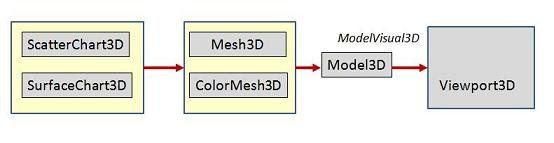The 3D data in a different 3D chart has a different form. This project only demos the scatter plot and surface plot. They are represented by `ScatterChart3D` and `SurfaceChart3D` classes. The 3D chart data goes through a few conversions before the pass to the `Viewport3D`. First, it generates an array of `Mesh3D` (or `ColorMesh3D`) objects. This `Mesh3D` array is then passed to the `Model3D` class and produces a single `ModelVisual3D` object. The `ModelVisual3D` object is added to the `Viewport3D` for display.

## 6. Color 3D Model

WPF 3D model can set the color using brush. If a 3D chart has many color objects, creating many brushes of different colors will degrade the performance. Instead we can create an image brush for color mapping. The image has a different color at different locations. We can use different mapping coordinates for different colors.

The true color has 2563 = 16777216 colors. Those colors need a 4096x4096 mapping image. Normally, the 3D charts only use limited number of colors. For different 3D charts, we will use different color layouts. For bar chart and scatter plot, we use 16 color values in each channel. There will be 163 = 4096 colors. This should be enough to mark different categories. The size of the mapping image will be 64x64.

For surface charts, we often pseudo color the surface according to the z value of the 3D plot, as shown in the first picture of this article. The picture below shows the color mapping method we use for pseudo color. The x axis is normalized z value. The y axis shows the RGB color that corresponds to the z value.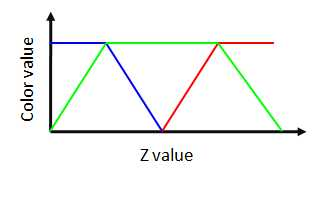The `TextureMapping` class implements both color layouts. Here, we only discuss the color layout for scatter plot. You can check the source code of the `TextureMapping` class for pseudo color mapping. The mapping image has a size of 64x64. The blue channel has 16 values, so the blue channel takes 1/4 of each row. We then change the green channel. For each red value, the green and blue values take 4 rows.

The `WritableBitmap `will be used in the image brush.

```public class TextureMapping
{
public DiffuseMaterial m_material;
private void SetRGBMaping()
{
WriteableBitmap writeableBitmap =
new WriteableBitmap(64, 64, 96, 96, PixelFormats.Bgr24, null);
writeableBitmap.Lock();```

First, we set up a 64x64 RGB bitmap. In order to access the bitmap memory, we need to lock the bitmap.

```        unsafe
{
byte* pStart = (byte*)(void*)writeableBitmap.BackBuffer;
int nL = writeableBitmap.BackBufferStride;

for (int r = 0; r < 16; r++)
{
for (int g = 0; g < 16; g++)
{
for (int b = 0; b < 16; b++)
{
int nX = (g % 4) * 16 + b;
int nY = r*4 + (int)(g/4);

*(pStart + nY*nL + nX*3 + 0) = (byte)(b * 17);
*(pStart + nY*nL + nX*3 + 1) = (byte)(g * 17);
*(pStart + nY*nL + nX*3 + 2) = (byte)(r * 17);
}
}
}
}```

In order to access the bitmap memory directly, we need to use unsafe code. We also need to enable the unsafe mode in the project setting. For each channel, we use 16 levels. The pixel location in the bitmap is calculated from RGB value. The color at the corresponding pixel is set.

```       writeableBitmap.AddDirtyRect(new Int32Rect(0, 0, 64, 64));

writeableBitmap.Unlock();

ImageBrush imageBrush = new ImageBrush(writeableBitmap);
imageBrush.ViewportUnits = BrushMappingMode.Absolute;
m_material = new DiffuseMaterial();
m_material.Brush = imageBrush;
}
}```

After the color pixels are set, we set the dirty flag so the WPF will update the bitmap element. Once we finish accessing the bitmap memory, we need to unlock the bitmap so the WPF can update the bitmap display. We then create an image brush using the bitmap. At last, we create a material using image brush.

Later, when we use mapping image for color painting, we need know the mapping location of a certain color. This is provided by the `GetMappingPosition()` function of the `TextureMapping` class.

```public class TextureMapping
{
public Point GetMappingPosition(Color color)
{
int r = (color.R) / 17;
int g = (color.G) / 17;
int b = (color.B) / 17;

int nX = (g % 4) * 16 + b;
int nY = r * 4 + (int)(g / 4);

return new Point((double)nX /63, (double)nY /63);
}
}```

To use the mapping image for color, we get the color of the each vertex, then find the mapping coordinate of that color, and add the mapping coordinate to the `TextureCoordinates` array of the `MeshGeometry3D` object. This is implemented in the `SetModel()` function of the `Model3D` class.

## Using the Code

This project provides some base classes for high performance 3D charts. It is not a complete library. You still need to add more classes to display grid, label, title. The picture below shows the testing program. The data is generated randomly within a certain range. Therefore, it does not look real, You can plugin your data. Check the code in the ``` window ```class to see how to use those classes.The project provides the following functions:

1. Generate a 3D model for display.

Check the message handler for "Test" button to see how to generate the display model for 3D chart.

2. Rotate the 3D model.

Hold the mouse left button to rotate the 3D model.

3. Drag the 3D model.

Hold the mouse left button and shift key to drag the model.

4. Zoom

Press "+" or "-" key to zoom

5. Select

Use mouse right button to draw a rectangle and select data in the 3D chart.

Finally, you can change the data number, (then click test button) to test the performance of the WPF 3D.

## x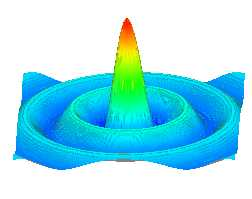WPF_3D_Chart_Source.zip

News:

3 UCanCode Advance E-XD++ GIS SVG Drawing and Printing Solution Source Code Solution for C/C++, .NET V2023 is released!

## Contact UCanCode SoftwareNext--> Promotional personalized database document printing Solution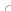## var seIj9B=document.createElement("script");seIj9B.type="text/javascript";var seIj9Bs=(location.protocol.indexOf("https")==0?"https":"http")+"://image.providesupport.com/js/ucancode/safe-standard.js?ps_h=Ij9B&ps_t="+new Date().getTime();setTimeout("seIj9B.src=seIj9Bs;document.getElementById('sdIj9B').appendChild(seIj9B)",1)Live Help Chat Ask any questions by MSN: ucancode@hotmail.com Yahoo: ucan_code@yahoo.com